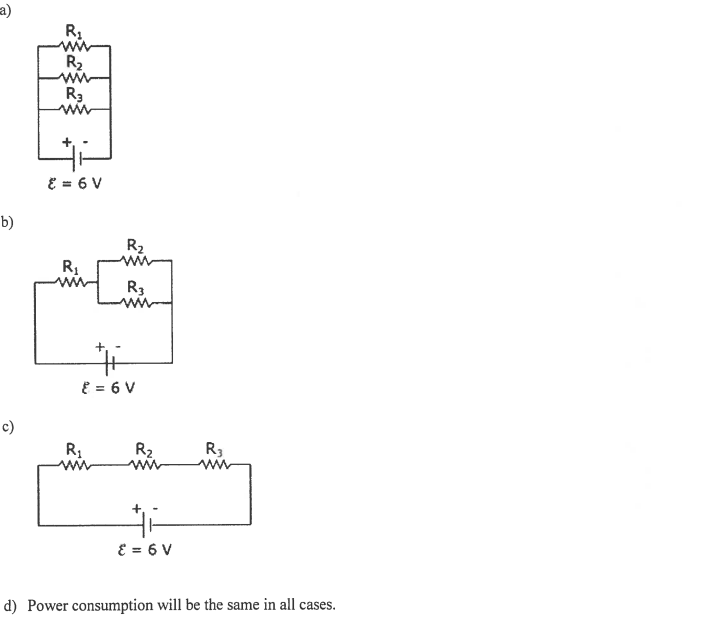# Problem: You have a battery supplying emf ε = 6V and negligible internal resistance. You also have three resistors with values R1 = 100 Ω, R2 = 200 Ω, and R3 = 400 Ω. What resistor combination will minimize power consumption?

###### FREE Expert Solution
81% (342 ratings)
###### Problem Details

You have a battery supplying emf ε = 6V and negligible internal resistance. You also have three resistors with values R1 = 100 Ω, R2 = 200 Ω, and R3 = 400 Ω. What resistor combination will minimize power consumption?